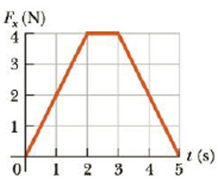Chapter 6, Problem 16P

Chapter
Section
Textbook Problem

A force of magnitude Fx acting in the x-direction on a 2.00-kg particle varies in time as shown in Figure P6.16. Find (a) the impulse of the force, (b) the final velocity of the particle if it is initially at rest, and (c) the final velocity of the particle if it is initially moving along the x-axis with a velocity of −2.00 m/s.Figure P6.16

(a)

To determine
The Impulse of the force.

Explanation

Given Information: The mass of the particle is m=2.0kg .

The expression for impulse is,

I=2Atri+Arec

• Atri is the area of the triangle,
• Arec is the area of the rectangle,
• I is the impulse,

Rewrite the above expression as

I=2(hw)rec+12(ba)tri (1)

• h is the height,
• w is the width
• b is the base,
• a is the altitude,

Substitute 4

(b)

To determine
The final velocity of the particle initially at rest

(c)

To determine
Final velocity of the particle initially moving along x-axis

Still sussing out bartleby?

Check out a sample textbook solution.

See a sample solution

The Solution to Your Study Problems

Bartleby provides explanations to thousands of textbook problems written by our experts, many with advanced degrees!

Get Started

Find more solutions based on key concepts